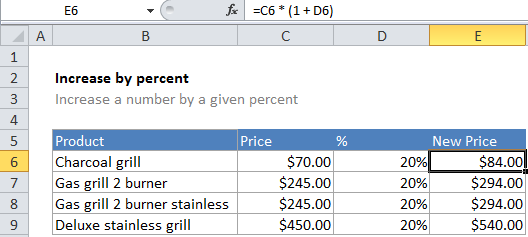# How to increase by percentage in Excel

If you need to increase a number by a certain percentage, you can use a simple formula that multiplies the number times the percent + 1.

## Formula

`=number*(1+percent)`## Explanation

### How this formula works

In the example, the active cell contains this formula:

`=C6*(1+D6)`

In this case, Excel first calculates the result of 1 + the value in D6 (.2) to get 1.2 which it then multiplies times the value in C6 (70) to get a final result of 84:

Worked Example:   How to Copy, Paste and Retain Exact Copied Formula in another Cell in Excel

=70 * (1 + .2)
=70 * (1.2)
=84

Worked Example:   Sum Largest Numbers using LARGE function in Excel

Note: you must add 1 to the percent if you want to increase a number. If you don’t, you’ll get the amount of the number the percentage represents.

Worked Example:   How to calculate percent variance in Excel

### All-in-one solution

If you want an all-in-one solution, you can use a formula like this to increase a value by 5%:

`=A1*105%`

Just change the percentage value to suit your needs.